GeometrizeTwitterBot  1.0 Python Twitter bot for geometrizing images into geometric primitives
tweet_parser Namespace Reference

This module contains code that reads tweet text and figures out how the bot should do in response. More...

Functions

def _represents_int (s)
Returns true if the given string represents an integer value. More...

def _clamp (n, smallest, largest)
Clamps the given number within the range smallest, largest. More...

Adds the given items to the given dictionary, mapping each keyword to the given shape name. More...

def _make_shape_keyword_dictionary ()
Creates a dictionary mapping shape keywords to canonical shape names used by ChaiScript scripts Provides a convenient way to map words in tweets like "rects" to the shape type "RECTANGLE". More...

def _max_quantity_for_shape_type (shape_type)
Gets the maximum number of shapes allowed for the given shape type This to to set a sensible limit on the length of time the bot will spend on a single image. More...

def _plural_name_for_shape_type (shape_type)
Gets a human-readable name for the given shape type. More...

def _make_loop_body (shape_type)
Helper for constructing a ChaiScript script for geometrizing shapes. More...

def _make_for_loop (step_count, shape_type)
Helper for constructing a ChaiScript script for geometrizing shapes. More...

def _make_shape_code (dict)
Helper for constructing a ChaiScript script for geometrizing shapes. More...

def make_shape_quantity_dictionary (message)
Parses a tweet message and returns a dictionary of the shape types and quantities that were requested. More...

def make_code_for_shape_tweet (message)
Parses the given tweet, returning the ChaiScript code for Geometrize to run based on the contents of the tweet. More...

def make_message_for_shape_dictionary (shape_quantity_dictionary)
Creates a message for the given shape dictionary, suitable for tweeting along with the result of running the script. More...

Variables

list _rect_keywords = [ "rect", "rects", "rectangle", "rectangles" ]

list _rotated_rect_keywords = [ "rotated_rect", "rotated_rects", "rotated_rectangle", "rotated_rectangles" ]

list _triangle_keywords = [ "tri", "tris", "triangle", "triangles" ]

list _ellipse_keywords = [ "ellipse", "ellipses" ]

list _rotated_ellipse_keywords = [ "rotated_ellipse", "rotated_ellipses" ]

list _circle_keywords = [ "circ", "circs", "circle", "circles" ]

list _line_keywords = [ "line", "lines" ]

list _polyline_keywords = [ "polyline", "polylines" ]

Detailed Description

This module contains code that reads tweet text and figures out how the bot should do in response.

Function Documentation

 def tweet_parser._add_shape_type_for_keywords ( dict, shape_keywords, shape_name )
private

Adds the given items to the given dictionary, mapping each keyword to the given shape name.

27  for shape_keyword in shape_keywords:
28  dict[shape_keyword] = shape_name
29
Here is the caller graph for this function: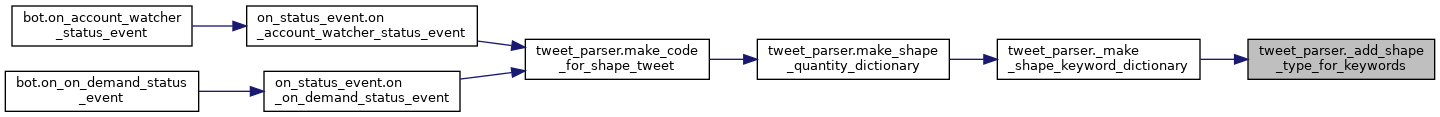◆ _clamp()

 def tweet_parser._clamp ( n, smallest, largest )
private

Clamps the given number within the range smallest, largest.

22 def _clamp(n, smallest, largest):
23  return max(smallest, min(n, largest))
24

◆ _make_for_loop()

 def tweet_parser._make_for_loop ( step_count, shape_type )
private

Helper for constructing a ChaiScript script for geometrizing shapes.

81 def _make_for_loop(step_count, shape_type):
82  return "for(var i = 0; i < " + str(step_count) + "; ++i) { " + _make_loop_body(shape_type) + " }";
83
Here is the call graph for this function: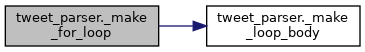Here is the caller graph for this function: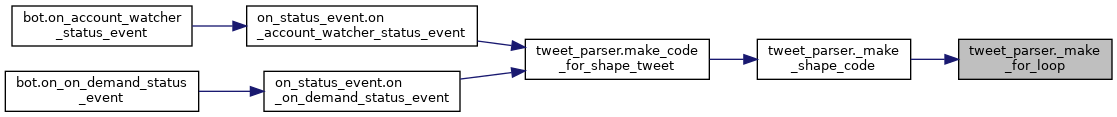◆ _make_loop_body()

 def tweet_parser._make_loop_body ( shape_type )
private

Helper for constructing a ChaiScript script for geometrizing shapes.

77 def _make_loop_body(shape_type):
79
Here is the caller graph for this function: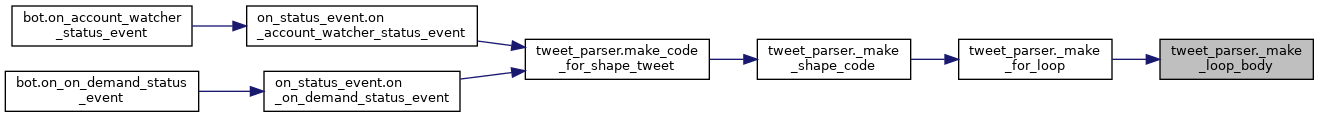◆ _make_shape_code()

 def tweet_parser._make_shape_code ( dict )
private

Helper for constructing a ChaiScript script for geometrizing shapes.

85 def _make_shape_code(dict):
86  code = ""
87  for key, value in dict.items():
88  code += _make_for_loop(value, key)
89  return code
90
Here is the call graph for this function: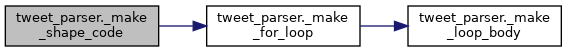Here is the caller graph for this function: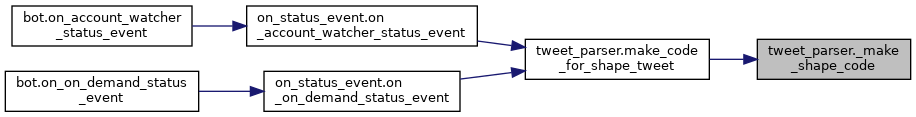◆ _make_shape_keyword_dictionary()

 def tweet_parser._make_shape_keyword_dictionary ( )
private

Creates a dictionary mapping shape keywords to canonical shape names used by ChaiScript scripts Provides a convenient way to map words in tweets like "rects" to the shape type "RECTANGLE".

33  dict = {}
43  return dict
44
Here is the call graph for this function: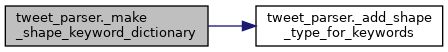Here is the caller graph for this function: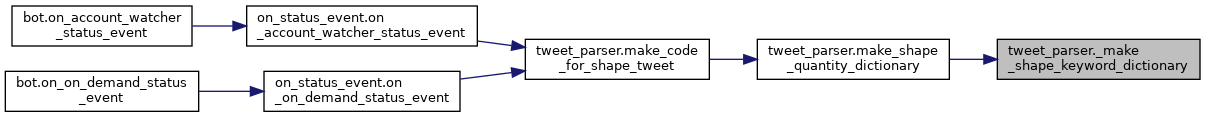◆ _max_quantity_for_shape_type()

 def tweet_parser._max_quantity_for_shape_type ( shape_type )
private

Gets the maximum number of shapes allowed for the given shape type This to to set a sensible limit on the length of time the bot will spend on a single image.

47 def _max_quantity_for_shape_type(shape_type):
48  dict = {}
49  dict["RECTANGLE"] = 500
50  dict["ROTATED_RECTANGLE"] = 750
51  dict["TRIANGLE"] = 750
52  dict["ELLIPSE"] = 750
53  dict["ROTATED_ELLIPSE"] = 750
54  dict["CIRCLE"] = 750
55  dict["LINE"] = 4000
57  dict["POLYLINE"] = 4000
58
59  return dict[shape_type]
60
Here is the caller graph for this function: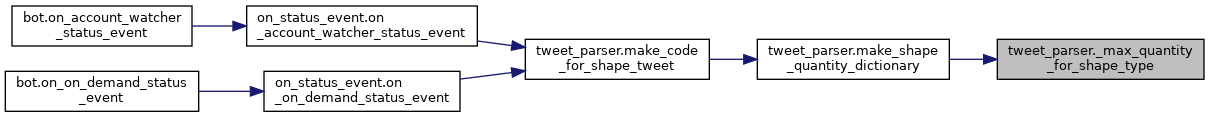◆ _plural_name_for_shape_type()

 def tweet_parser._plural_name_for_shape_type ( shape_type )
private

Gets a human-readable name for the given shape type.

62 def _plural_name_for_shape_type(shape_type):
63  dict = {}
64  dict["RECTANGLE"] = "Rectangles"
65  dict["ROTATED_RECTANGLE"] = "Rotated Rectangles"
66  dict["TRIANGLE"] = "Triangles"
67  dict["ELLIPSE"] = "Ellipses"
68  dict["ROTATED_ELLIPSE"] = "Rotated Ellipses"
69  dict["CIRCLE"] = "Circles"
70  dict["LINE"] = "Lines"
72  dict["POLYLINE"] = "Polylines"
73
74  return dict[shape_type]
75
Here is the caller graph for this function: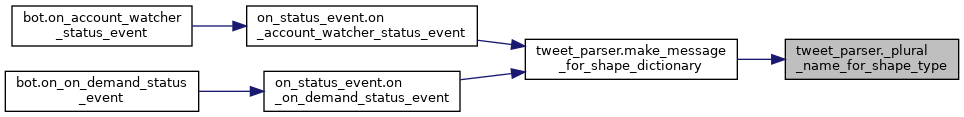◆ _represents_int()

 def tweet_parser._represents_int ( s )
private

Returns true if the given string represents an integer value.

18 def _represents_int(s):
19  return re.match(r"[-+]?\d+\$", s) is not None
20
Here is the caller graph for this function: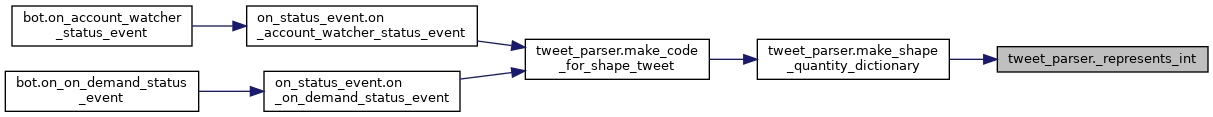◆ make_code_for_shape_tweet()

 def tweet_parser.make_code_for_shape_tweet ( message )

Parses the given tweet, returning the ChaiScript code for Geometrize to run based on the contents of the tweet.

The shapes are added in the order they are specified in the message. Message examples: "@Geometrizer triangles=30 and rectangles=20, thanks bot!" - produces an image with 30 triangles, then 20 rectangles. Or: "@Geometrizer triangles=30 circles=500, triangles=20, nice bot!" - produces an image with 30 triangles, 500 circles, 20 triangles. Or: "@Geometrizer tris=300 circs=200" - produces an image with 300 triangles, 200 circles. Or: "@Geometrize rotated_rects=500" - produces an image with 500 rotated rectangles. Or: "@Geometrizer this is a really cool bot!" - produces an image with a random selection and quantity of shapes. :return A chunk of ChaiScript code that adds the shapes that the tweet requests (must be combined with a larger script to work).

134 def make_code_for_shape_tweet(message):
135
136  shape_quantity_dictionary = make_shape_quantity_dictionary(message)
137  if shape_quantity_dictionary:
138  # Try to match shapeTypeN=shapeQuantityN patterns
139  code = _make_shape_code(shape_quantity_dictionary)
140  print("Creating specific shapes and quantities code for tweet")
141  return code, shape_quantity_dictionary
142
143  # Failed, so use a random shape type instead
144  print("Creating random shapes code for tweet")
145
146  # Use a subset of possible shapes since random numbers of others don't always look good
147  shape_types = ['ROTATED_RECTANGLE', 'ROTATED_ELLIPSE', 'TRIANGLE', 'CIRCLE', 'ELLIPSE']
148  shape_type = random.choice(shape_types)
149  shape_quantity = random.randint(200, 500)
150  shape_quantity_dictionary[shape_type] = shape_quantity
151  code = _make_shape_code(shape_quantity_dictionary)
152
153  return code, shape_quantity_dictionary
154
Here is the call graph for this function: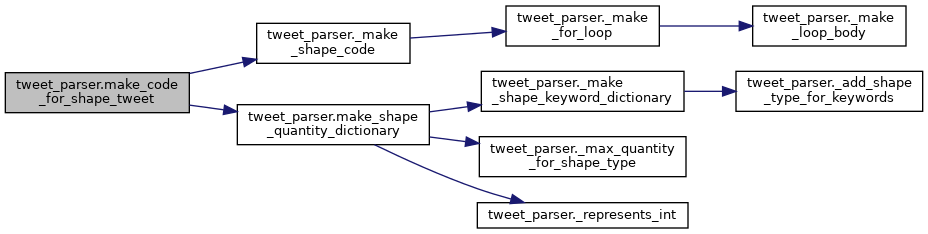Here is the caller graph for this function: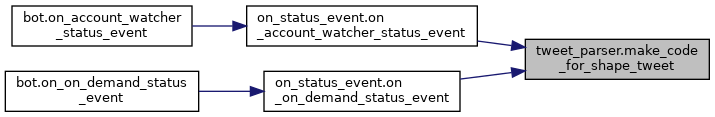◆ make_message_for_shape_dictionary()

 def tweet_parser.make_message_for_shape_dictionary ( shape_quantity_dictionary )

Creates a message for the given shape dictionary, suitable for tweeting along with the result of running the script.

For example [ "ROTATED_RECTANGLES" => 250 ], "250 Rotated Rectangles" :return A message describing the shapes and quantities.

158 def make_message_for_shape_dictionary(shape_quantity_dictionary):
159  message = "Geometrized - "
160
161  for shape, quantity in shape_quantity_dictionary.items():
162  message += str(quantity)
163  message += " "
164  message += _plural_name_for_shape_type(shape)
165  message += " "
166
167  return message
Here is the call graph for this function: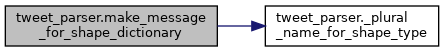Here is the caller graph for this function: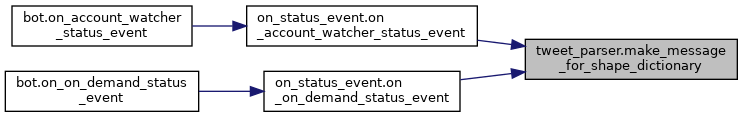◆ make_shape_quantity_dictionary()

 def tweet_parser.make_shape_quantity_dictionary ( message )

Parses a tweet message and returns a dictionary of the shape types and quantities that were requested.

93  symbols = message.split(" ")
94  shape_keyword_dictionary = _make_shape_keyword_dictionary()
95  shape_quantity_dictionary = {}
96
97  for symbol in symbols:
98  pair = symbol.split("=")
99
100  if len(pair) != 2:
101  continue
102
103  shape_type_key = pair.strip()
104  if shape_type_key not in shape_keyword_dictionary:
105  continue
106
107  if not _represents_int(pair.strip()):
108  continue
109
110  shape_type = shape_keyword_dictionary[shape_type_key]
111  shape_count = int(pair.strip())
112  shape_count_max = _max_quantity_for_shape_type(shape_type)
113
114  if shape_count <= 0:
115  print("Got bad shape count request, must be positive")
116  continue
117
118  if shape_count >= shape_count_max:
119  print("Requested shape count was too high, clamping it down")
120  shape_count = shape_count_max
121
122  shape_quantity_dictionary[shape_type] = shape_count
123
124  return shape_quantity_dictionary
125
Here is the call graph for this function: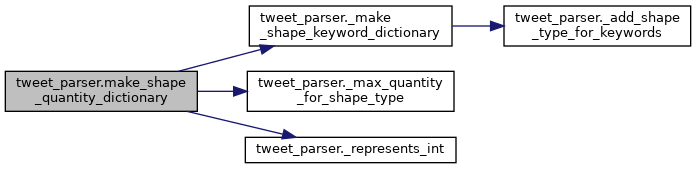Here is the caller graph for this function: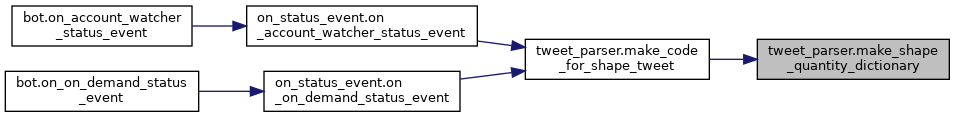◆ _circle_keywords

 list tweet_parser._circle_keywords = [ "circ", "circs", "circle", "circles" ]
private

◆ _ellipse_keywords

 list tweet_parser._ellipse_keywords = [ "ellipse", "ellipses" ]
private

◆ _line_keywords

 list tweet_parser._line_keywords = [ "line", "lines" ]
private

◆ _polyline_keywords

 list tweet_parser._polyline_keywords = [ "polyline", "polylines" ]
private

private

◆ _rect_keywords

 list tweet_parser._rect_keywords = [ "rect", "rects", "rectangle", "rectangles" ]
private

◆ _rotated_ellipse_keywords

 list tweet_parser._rotated_ellipse_keywords = [ "rotated_ellipse", "rotated_ellipses" ]
private

◆ _rotated_rect_keywords

 list tweet_parser._rotated_rect_keywords = [ "rotated_rect", "rotated_rects", "rotated_rectangle", "rotated_rectangles" ]
private

◆ _triangle_keywords

 list tweet_parser._triangle_keywords = [ "tri", "tris", "triangle", "triangles" ]
private
tweet_parser._make_shape_keyword_dictionary
def _make_shape_keyword_dictionary()
Creates a dictionary mapping shape keywords to canonical shape names used by ChaiScript scripts Provi...
Definition: tweet_parser.py:32
tweet_parser._make_for_loop
def _make_for_loop(step_count, shape_type)
Helper for constructing a ChaiScript script for geometrizing shapes.
Definition: tweet_parser.py:81
tweet_parser._plural_name_for_shape_type
def _plural_name_for_shape_type(shape_type)
Gets a human-readable name for the given shape type.
Definition: tweet_parser.py:62
tweet_parser._clamp
def _clamp(n, smallest, largest)
Clamps the given number within the range smallest, largest.
Definition: tweet_parser.py:22
tweet_parser._represents_int
def _represents_int(s)
Returns true if the given string represents an integer value.
Definition: tweet_parser.py:18
Adds the given items to the given dictionary, mapping each keyword to the given shape name.
Definition: tweet_parser.py:26
tweet_parser._make_loop_body
def _make_loop_body(shape_type)
Helper for constructing a ChaiScript script for geometrizing shapes.
Definition: tweet_parser.py:77
tweet_parser.make_shape_quantity_dictionary
def make_shape_quantity_dictionary(message)
Parses a tweet message and returns a dictionary of the shape types and quantities that were requested...
Definition: tweet_parser.py:92
tweet_parser._make_shape_code
def _make_shape_code(dict)
Helper for constructing a ChaiScript script for geometrizing shapes.
Definition: tweet_parser.py:85
tweet_parser.make_code_for_shape_tweet
def make_code_for_shape_tweet(message)
Parses the given tweet, returning the ChaiScript code for Geometrize to run based on the contents of ...
Definition: tweet_parser.py:134
tweet_parser._max_quantity_for_shape_type
def _max_quantity_for_shape_type(shape_type)
Gets the maximum number of shapes allowed for the given shape type This to to set a sensible limit on...
Definition: tweet_parser.py:47
tweet_parser.make_message_for_shape_dictionary
def make_message_for_shape_dictionary(shape_quantity_dictionary)
Creates a message for the given shape dictionary, suitable for tweeting along with the result of runn...
Definition: tweet_parser.py:158